# Dinosaurs

More than 30 and less than 60 dinosaurs have met at the pond. A quarter of them bathed and 1/7 saws and the rest gripped. How many were at the pond? How many were there?

Result

n =  56
j =  8

#### Solution:Leave us a comment of example and its solution (i.e. if it is still somewhat unclear...):

Showing 0 comments:Be the first to comment!#### To solve this example are needed these knowledge from mathematics:

Do you want to calculate least common multiple two or more numbers?

## Next similar examples:

1. Lesson exercising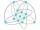The lesson of physical education, pupils are first divided into three groups so that each has the same number. The they redistributed, but into six groups. And again, it was the same number of children in each group. Finally they divided into nine equal gr
2. Cents no more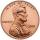Janko bought pencils for 35 cents each. Neither he nor the salesperson had small coins just a whole € 1 coin. At least how many pencils had to buy to pay for the whole euros?
3. School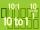Headteacher think whether the distribution of pupils in race in groups of 4,5,6,9 or 10. How many pupils must have at least school at possible options?
4. Balls groups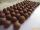Karel pulled the balls out of his pocket and divide it into the groups. He could divide them in four, six or seven, and no ball ever left. How little could be a ball?
5. Matches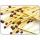George poured out of the box matches and composing them triangles and no match was left. Then he tries squares, hexagons and octagons and no match was left. How many matches must be at least in the box?
6. The smallest numberWhat is the smallest number that can be divided by both 5 and 7
7. Lcm of three numbersWhat is the Lcm of 120 15 and 5
8. Addition of Roman numbersAdded together and write as decimal number: LXVII + MLXIV
9. Lcm simpleFind least common multiple of this two numbers: 140 175.
10. ProductResult of the product of the numbers 1, 2, 3, 1, 2, 0 is:
11. Roman numeralsWrite numbers written in Roman numerals as decimal.
12. Write decimalsWrite in the decimal system the short and advanced form of these numbers: a) four thousand seventy-nine b) five hundred and one thousand six hundred and ten c) nine million twenty-six
13. Counting numberWhat is the smallest counting number divisible by 2,5,7,8 and 15?
14. LCMWhat is the least common multiple of 36, 44, 21?
15. 3 buses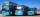At morning 5:00 am three buses started from one place. The first travel in five-minute intervals, the second at 10-minute intervals and the third at 25-minute intervals. At what hour will again be the three buses coming from the same place?
16. Three buses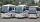Three public transport buses depart together from the bus station in the morning. The first bus was returning to the station after 18 minutes, the second after 12 minutes and a third after 24 minutes. How long will again together on the station? Result exp
17. The port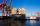Four ships Berthed in the port. Together sail from a port. The first ship will return to port every two weeks, second ship after 4 weeks, third after 8 weeks and fourth ship after 12 weeks. After how many weeks all the boats gather at the port?